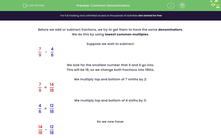# Use Common Multiples to Find Common Denominators

In this worksheet, students will use common multiples to express fractions in the same denominators.Key stage:  KS 2

Curriculum topic:   Number: Fractions, Decimals and Percentages

Popular topics:   Fractions worksheets

Difficulty level:#### Worksheet Overview

Before we add or subtract fractions, we try to get them to have the same denominators.

We do this by using lowest common multiples.

Suppose we wish to subtract:

 7 - 4 9 6

We look for the smallest number that 9 and 6 go into.

This will be 18, so we change both fractions into 18ths.

We multiply both the numerator and the denominator of 7/9 by 2:

 7 = 14 9 18

We multiply the numerator and the denominator of 4/6 by 3:

 4 = 12 6 18

So we now have:

 14 - 12 18 18

 2 = 1 18 9

Example

When we work out this calculation, what is the lowest common denominator we should choose for both fractions?

 7 - 4 15 10

The lowest common multiple of 15 and 10 is 30, because 2 × 15 = 30 and 3 × 10 = 30.

We need to multiply both the numerator and denominator of 7/15 by 2, which gives us 14/30

We need to multiply both the numerator and denominator of 4/10 by 3, which gives us 12/30

14/30 - 12/30 = 2/30 which can be simplified to 1/15.

Now it's time to try some questions.

### What is EdPlace?

We're your National Curriculum aligned online education content provider helping each child succeed in English, maths and science from year 1 to GCSE. With an EdPlace account you’ll be able to track and measure progress, helping each child achieve their best. We build confidence and attainment by personalising each child’s learning at a level that suits them.

Get started#### Popular Maths topics

••••